# Wave-Pinning

##### Persistent Identifier

Spatial distribution of GTPase activity

## Introduction

The actin cytoskeleton is regulated by a set of proteins called Small GTPases. The spatial distribution of GTPase activity changes on a timescale of seconds in rapidly moving cells like white blood cells (neutrophils). Here, we generalize a well-mixed GTPase model system to include spatial distribution.

In this context, we investigate the influence of the conservation of the total GTPase and discuss the different behaviors that occur in various domains such as

• traveling waves for a constant pool of inactive GTPase in 1D,
• stalling wave (wave-pinning) for constant total GTPase in 1D,
• stalling wave in a 2D rectangular domain,
• stalling wave in a 2D oval and irregular domain,
• effects of edge curvature on wave-pinning behavior in 2D domains.

## Description

Consider the equations depicting a GTPase model, choosing $u$ as it’s active and $v$ as the inactive form:

\begin{align} \frac{\partial u}{\partial t} &= (b + \gamma \frac{u^n}{1+u^n})v - u + D_u\Delta u \\ \frac{\partial v}{\partial t} &= - (b + \gamma \frac{u^n}{1+u^n})v + u + D_v\Delta v \\ \end{align}

## Results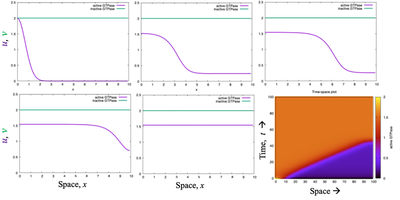A simulation of the GTPase model in 1D, with no-flux BoundaryConditions and a constant pool of inactive form $\nu = 2$. A wave that is initiated at the left boundary sweeps all the way across the domain so that the entire cell has a uniform GTPase level of activity (spatial profiles depicted at $t = 0, 15, 30, 45, 60$). Produced with the Morpheus file NotWavepinning_main.xml.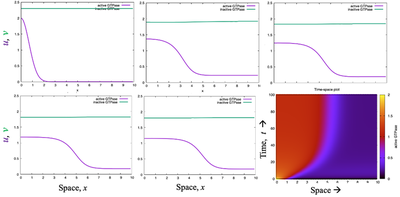As in the figure above, but for constant total GTPase (2 PDEs), demonstrating wave-pinning behavior. In contrast to the figure above, the waves only move part ways into the domain, so that a spatially nonuniform steady state is created. The right part of the 1D domain has low GTPase activity, whereas the left has high activity. The same result is shown in the kymograph (lower right panel). Produced with the Morpheus file WavePinning2PDEsConservedTotal1D.xml.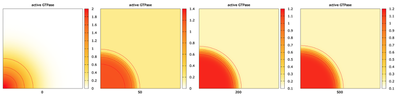A simulation of the wave-pinning GTPase model in 2D on a rectangular domain, stimulated at the lower left corner. Shown are the plots for $t = 0, 50, 200, 500$ time steps together with level curves of the level of active GTPase. The total GTPase is conserved. Produced with the Morpheus file Wavepinningin2D.xml.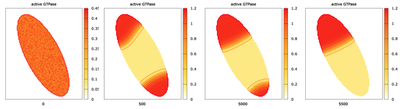A simulation of the wave-pinning GTPase model in 2D on an oval shaped domain, with random initial conditions and no-flux BoundaryConditions. Shown are the plots for $t = 0, 500, 5000, 5500$ with activated isolines (red). Produced with the Morpheus file Wavepinning2DOvalDomain.xml.

In the simulation shown in the figure above, the system quickly forms hotspots at the two opposite poles of the domain. These persist for a long time. One peak eventually wins and the other disappears.

The model Wavepinning2DOvalDomain.xml also requires the separate file Rodlarger.tiff.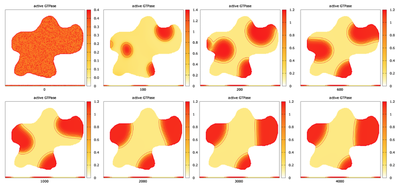A simulation of the wave-pinning GTPase model in an irregular domain with no-flux BoundaryConditions starting with random intial conditions. Shown is the level of active form and the level curves for GTPase for times $t = 0, 100, 200, 600$ (top row) and $t = 1000, 2000, 3000, 4000$ (bottom row) with isolines (red) enabled. Produced with the Morpheus file Wavepinningin2DIregDomain.xml.
The model Wavepinningin2DIregDomain.xml also requires the separate file domainLarge.tiff.

In the figure above, produced with the Morpheus file Wavepinningin2DIregDomain.xml, the intial dynamics quickly set up a few ‘hotspots’ that gradually merge and/or attach to the domain boundary. On a much slower time-scale, the high activity zones gradually evolve to minimize their interface length. The shape of the domain means that hotspots get ‘trapped’ in convex regions.

## Model

Get this model via:

•  Download: NotWavepinning_main.xml
• XML Preview

<MorpheusModel version="4">
<Description>
<Title>NotWavepinning</Title>
<Details>Full title:		Wave-Pinning
Authors:		L. Edelstein-Keshet
Contributors:	Y. Xiao
Date:		22.06.2022
Model ID:		https://identifiers.org/morpheus/M2011
File type:		Main model
Reference:		L. Edelstein-Keshet: Mathematical Models in Cell Biology
Comment:		Single GTPase model but with constant inactive GTPase. This simulation demonstrates that when there is no conservation of total (active plus inactive) GTPase, then there is no wave-pinning. The wave of activity takes over the entire domain. Credit: Lutz Brusch, for an earlier version of this code for the wave-pinning model with conservation.</Details>
</Description>
<Global>
<Constant symbol="L" value="dx*size.x"/>
<Function symbol="x">
<Expression>dx*space.x</Expression>
</Function>
<Field symbol="a" name="active GTPase" value="2*exp(-x^2)">
<Diffusion rate="0.1"/>
</Field>
<Field symbol="i" name="inactive GTPase" value="2">
<Diffusion rate="10"/>
</Field>
<DiffEqn symbol-ref="a">
<Expression> i*(b+gamma*a^n/(1+a^n))- a  </Expression>
</DiffEqn>
<DiffEqn symbol-ref="i">
<Expression>0</Expression>
</DiffEqn>
<Constant symbol="gamma" name="feedback activation parameter" value="1"/>
<Constant symbol="n" value="2"/>
<Function symbol="b" name="basal activation rate">
<Expression>0.067</Expression>
</Function>
</System>
</Global>
<Space>
<Lattice class="linear">
<Size symbol="size" value="100, 0, 0"/>
<BoundaryConditions>
<Condition type="noflux" boundary="x"/>
<Condition type="noflux" boundary="-x"/>
</BoundaryConditions>
<NodeLength symbol="dx" value="0.1"/>
<Neighborhood>
<Order>1</Order>
</Neighborhood>
</Lattice>
<SpaceSymbol symbol="space"/>
</Space>
<Time>
<StartTime value="0"/>
<StopTime value="100"/>
<TimeSymbol symbol="t" name="time"/>
</Time>
<Analysis>
<Logger time-step="1">
<Input>
<Symbol symbol-ref="a"/>
<Symbol symbol-ref="i"/>
<Symbol symbol-ref="L"/>
</Input>
<Output>
<TextOutput/>
</Output>
<Plots>
<Plot time-step="5" title="Spatial profiles">
<Style decorate="true" line-width="3.0" style="lines"/>
<Terminal terminal="png"/>
<X-axis>
<Symbol symbol-ref="x"/>
</X-axis>
<Y-axis maximum="2.5" minimum="0">
<Symbol symbol-ref="a"/>
<Symbol symbol-ref="i"/>
</Y-axis>
<Range>
<Time mode="current"/>
</Range>
</Plot>
<Plot time-step="-1" title="Time-space plot">
<Style style="points"/>
<Terminal terminal="png"/>
<X-axis>
<Symbol symbol-ref="space.x"/>
</X-axis>
<Y-axis>
<Symbol symbol-ref="t"/>
</Y-axis>
<Color-bar>
<Symbol symbol-ref="a"/>
</Color-bar>
</Plot>
</Plots>
</Logger>
<ModelGraph format="svg" reduced="false" include-tags="#untagged"/>
</Analysis>
</MorpheusModel>